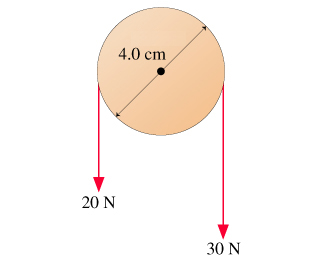# Problem: What is the magnitude of the net torque on the pulley about the axle?

###### FREE Expert Solution

Torque equations:

$\overline{){\mathbf{\tau }}{\mathbf{=}}{\mathbf{r}}{\mathbf{F}}{\mathbf{s}}{\mathbf{i}}{\mathbf{n}}{\mathbf{\theta }}}$ where θ is the angle between r and F.

The tension and the radius are perpendicular. Thus, θ = 90°.

86% (373 ratings)###### Problem Details

What is the magnitude of the net torque on the pulley about the axle?